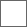• 12回复贴，共1

### 一起做道题目！A=100
B=70
C=30
~~~~~~~

b倒30到c里
c倒回a里30

……………………………………………

a的80倒c里，a里剩5030+30+30-70=20
20+30=50

A=100
B=70
C=30 A倒入C两次装入B A再倒入C 此时A=10 B=60 C=30 C把B装满 B再倒入A 此时A=80 B=0 C=20 C再倒入B A再倒入C A=50A=100
B=70
C=30
A倒满B，B两次倒满C并倒入A，

#### 扫二维码下载贴吧客户端